# 深度学习（四）卷积神经网络入门学习(1)

1、卷积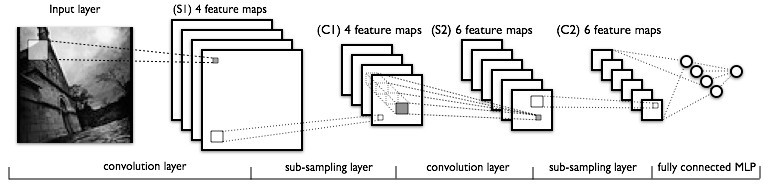2、池化

CNN的池化(图像下采样)方法很多：Mean pooling(均值采样)、Max pooling(最大值采样)、Overlapping (重叠采样)、L2 pooling(均方采样)、Local Contrast Normalization(归一化采样)、Stochasticpooling(随即采样)、Def-pooling(形变约束采样)。其中最经典的是最大池化，因此我就解释一下最大池化的实现：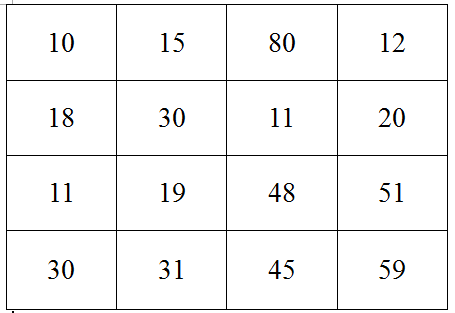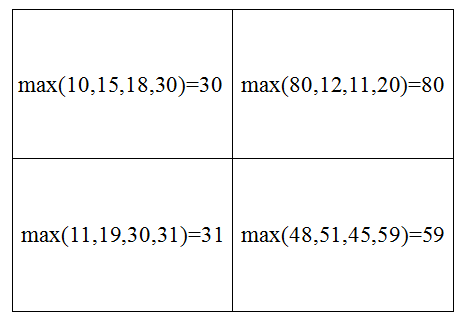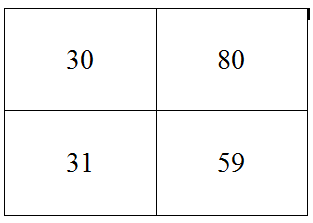3、feature maps

4、CNN的经典结构

(1)LeNet-5。这个是n多年前就有的一个CNN的经典结构，主要是用于手写字体的识别，也是刚入门需要学习熟悉的一个网络，我的这篇博文主要就是要讲这个网络(2)AlexNet。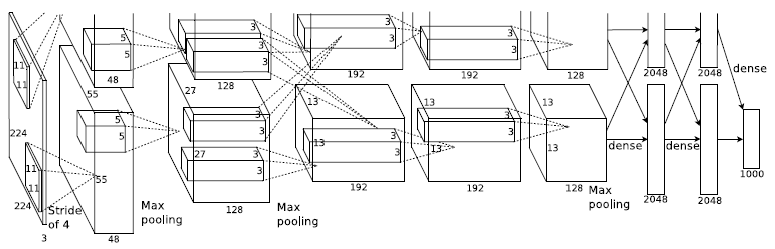OK，理论阶段就啰嗦到这里就好了，接着就讲解 LeNet-5， LeNet-5是用于手写字体的识别的一个经典CNN：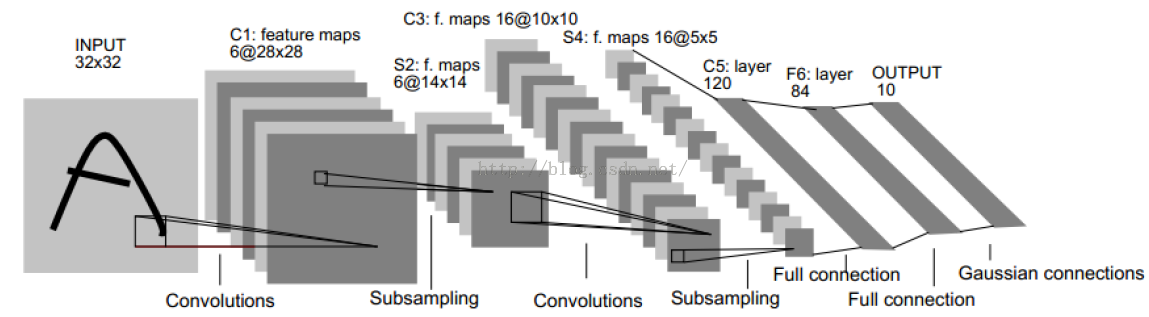LeNet-5结构

C1层：paper作者，选择6个特征卷积核，然后卷积核大小选择5*5，这样我们可以得到6个特征图，然后每个特征图的大小为32-5+1=28，也就是神经元的个数为6*28*28=784。

S2层：这就是下采样层，也就是使用最大池化进行下采样，池化的size，选择(2,2)，也就是相当于对C1层28*28的图片，进行分块，每个块的大小为2*2，这样我们可以得到14*14个块，然后我们统计每个块中，最大的值作为下采样的新像素，因此我们可以得到S1结果为：14*14大小的图片，共有6个这样的图片。

C3层：卷积层，这一层我们选择卷积核的大小依旧为5*5，据此我们可以得到新的图片大小为14-5+1=10，然后我们希望可以得到16张特征图。那么问题来了？这一层是最难理解的，我们知道S2包含：6张14*14大小的图片，我们希望这一层得到的结果是：16张10*10的图片。这16张图片的每一张，是通过S2的6张图片进行加权组合得到的，具体是怎么组合的呢？问题如下图所示：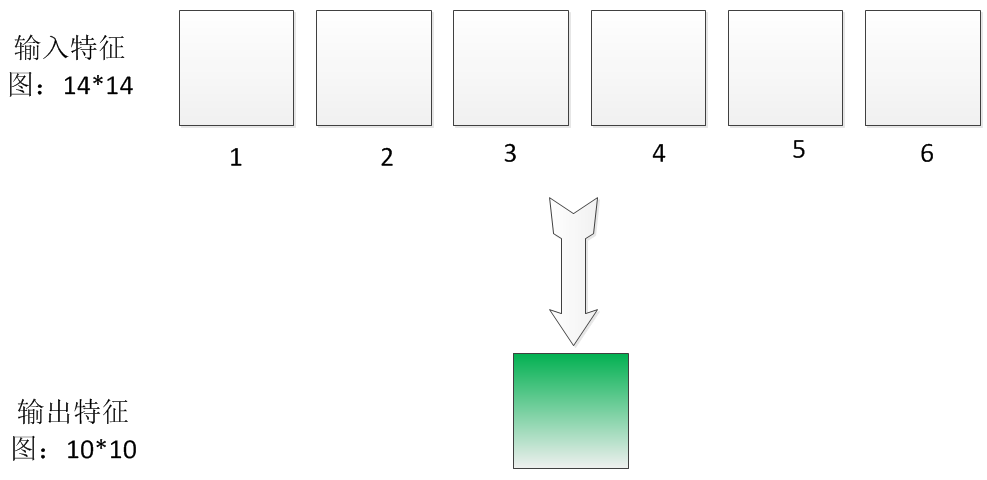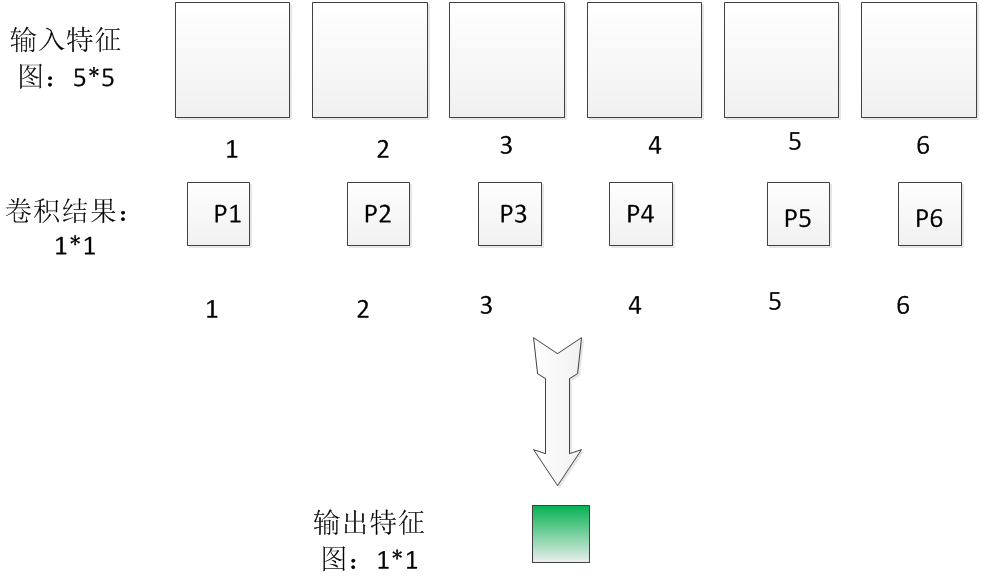为了简便起见，我这里先做一些标号的定义：我们假设输入第i个特征图的各个像素值为x1i，x2i……x25i，因为每个特征图有25个像素。因此第I个特征图经过5*5的图片卷积后，得到的卷积结果图片的像素值Pi可以表示成：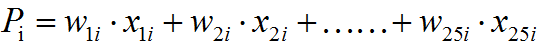P=P1+P2+……P6

P=WX

Out=f(P+b)

而我们希望得到16张10*10的输出特征图，因此我们就需要卷积参数个数为16*(6*(5*5))=16*6*(5*5)个参数。总之，C3层每个图片是通过S2图片进行卷积后，然后相加，并且加上偏置b,最后在进行激活函数映射得到的结果。

S4层：下采样层，比较简单，也是知己对C3的16张10*10的图片进行最大池化，池化块的大小为2*2。因此最后S4层为16张大小为5*5的图片。至此我们的神经元个数已经减少为：16*5*5=400。

C5层：我们继续用5*5的卷积核进行卷积，然后我们希望得到120个特征图。这样C5层图片的大小为5-5+1=1，也就是相当于1个神经元，120个特征图，因此最后只剩下120个神经元了。这个时候，神经元的个数已经够少的了，后面我们就可以直接利用全连接神经网络，进行这120个神经元的后续处理，后面具体要怎么搞，只要懂多层感知器的都懂了，不解释。

1、训练数据获取

<span style="font-size:18px;">import cPickle
import gzip
import numpy as np
import matplotlib.pyplot as plt
f = gzip.open('mnist.pkl.gz', 'rb')
train_set, valid_set, test_set = cPickle.load(f)
f.close()
tx,ty=train_set;

#查看训练样本
print np.shape(tx)#可以看到tx大小为（50000,28*28）的二维矩阵
print np.shape(ty)#可以看到ty大小为（50000,1）的矩阵
#图片显示
A=tx.reshape(28,28)#第八个训练样本
Y=ty
print Y
plt.imshow(A,cmap='gray')#显示手写字体图片</span>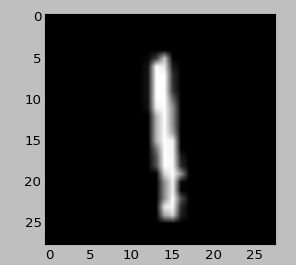2、LeNet-5实现

import os
import sys
import timeit

import numpy

import theano
import theano.tensor as T
from theano.tensor.signal import downsample
from theano.tensor.nnet import conv

from logistic_sgd import LogisticRegression, load_data
from mlp import HiddenLayer

#卷积神经网络的一层，包含：卷积+下采样两个步骤
#算法的过程是：卷积-》下采样-》激活函数
class LeNetConvPoolLayer(object):

#image_shape是输入数据的相关参数设置  filter_shape本层的相关参数设置
def __init__(self, rng, input, filter_shape, image_shape, poolsize=(2, 2)):
"""
:type rng: numpy.random.RandomState
:param rng: a random number generator used to initialize weights

3、input: 输入特征图数据，也就是n幅特征图片

4、参数 filter_shape: (number of filters, num input feature maps,
filter height, filter width)
num of filters：是卷积核的个数，有多少个卷积核，那么本层的out feature maps的个数
也将生成多少个。num input feature maps：输入特征图的个数。
然后接着filter height, filter width是卷积核的宽高，比如5*5,9*9……
filter_shape是列表，因此我们可以用filter_shape获取卷积核个数

5、参数 image_shape: (batch size, num input feature maps,
image height, image width)，
batch size：批量训练样本个数 ，num input feature maps：输入特征图的个数
image height, image width分别是输入的feature map图片的大小。
image_shape是一个列表类型，所以可以直接用索引，访问上面的4个参数，索引下标从
0~3。比如image_shape=image_heigth  image_shape=num input feature maps

6、参数 poolsize: 池化下采样的的块大小，一般为(2,2)
"""

assert image_shape == filter_shape#判断输入特征图的个数是否一致，如果不一致是错误的
self.input = input

# fan_in=num input feature maps *filter height*filter width
#numpy.prod(x)函数为计算x各个元素的乘积
#也就是说fan_in就相当于每个即将输出的feature  map所需要链接参数权值的个数
fan_in = numpy.prod(filter_shape[1:])
# fan_out=num output feature maps * filter height * filter width
fan_out = (filter_shape * numpy.prod(filter_shape[2:]) /
numpy.prod(poolsize))
# 把参数初始化到[-a,a]之间的数，其中a=sqrt(6./(fan_in + fan_out)),然后参数采用均匀采样
#权值需要多少个？卷积核个数*输入特征图个数*卷积核宽*卷积核高？这样没有包含采样层的链接权值个数
W_bound = numpy.sqrt(6. / (fan_in + fan_out))
self.W = theano.shared(
numpy.asarray(
rng.uniform(low=-W_bound, high=W_bound, size=filter_shape),
dtype=theano.config.floatX
),
borrow=True
)

# b为偏置，是一维的向量。每个输出特征图i对应一个偏置参数b[i]
#,因此下面初始化b的个数就是特征图的个数filter_shape
b_values = numpy.zeros((filter_shape,), dtype=theano.config.floatX)
self.b = theano.shared(value=b_values, borrow=True)

# 卷积层操作，函数conv.conv2d的第一个参数为输入的特征图，第二个参数为随机出事化的卷积核参数
#第三个参数为卷积核的相关属性，输入特征图的相关属性
conv_out = conv.conv2d(
input=input,
filters=self.W,
filter_shape=filter_shape,
image_shape=image_shape
)

# 池化操作，最大池化
pooled_out = downsample.max_pool_2d(
input=conv_out,
ds=poolsize,
ignore_border=True
)
#激励函数，也就是说是先经过卷积核再池化后，然后在进行非线性映射
# add the bias term. Since the bias is a vector (1D array), we first
# reshape it to a tensor of shape (1, n_filters, 1, 1). Each bias will
# thus be broadcasted across mini-batches and feature map
# width & height
self.output = T.tanh(pooled_out + self.b.dimshuffle('x', 0, 'x', 'x'))

# 保存参数
self.params = [self.W, self.b]
self.input = input

#测试函数
def evaluate_lenet5(learning_rate=0.1, n_epochs=200,
dataset='mnist.pkl.gz',
nkerns=[20, 50], batch_size=500):
""" Demonstrates lenet on MNIST dataset

:learning_rate: 梯度下降法的学习率

:n_epochs: 最大迭代次数

:type dataset: string
:param dataset: path to the dataset used for training /testing (MNIST here)

:nkerns: 每个卷积层的卷积核个数，第一层卷积核个数为 nkerns=20,第二层卷积核个数
为50个
"""

rng = numpy.random.RandomState(23455)

train_set_x, train_set_y = datasets#训练数据
valid_set_x, valid_set_y = datasets#验证数据
test_set_x, test_set_y = datasets#测试数据

# 计算批量训练可以分多少批数据进行训练，这个只要是知道批量训练的人都知道
n_train_batches = train_set_x.get_value(borrow=True).shape#训练数据个数
n_valid_batches = valid_set_x.get_value(borrow=True).shape
n_test_batches = test_set_x.get_value(borrow=True).shape
n_train_batches /= batch_size#批数
n_valid_batches /= batch_size
n_test_batches /= batch_size

# allocate symbolic variables for the data
index = T.lscalar()  # index to a [mini]batch

# start-snippet-1
x = T.matrix('x')   # the data is presented as rasterized images
y = T.ivector('y')  # the labels are presented as 1D vector of
# [int] labels

# Reshape matrix of rasterized images of shape (batch_size, 28 * 28)
# to a 4D tensor, compatible with our LeNetConvPoolLayer
# (28, 28) is the size of MNIST images.
layer0_input = x.reshape((batch_size, 1, 28, 28))

'''构建第一层网络：
image_shape：输入大小为28*28的特征图，batch_size个训练数据，每个训练数据有1个特征图
filter_shape：卷积核个数为nkernes=20，因此本层每个训练样本即将生成20个特征图
经过卷积操作，图片大小变为(28-5+1 , 28-5+1) = (24, 24)
经过池化操作，图片大小变为 (24/2, 24/2) = (12, 12)
最后生成的本层image_shape为(batch_size, nkerns, 12, 12)'''
layer0 = LeNetConvPoolLayer(
rng,
input=layer0_input,
image_shape=(batch_size, 1, 28, 28),
filter_shape=(nkerns, 1, 5, 5),
poolsize=(2, 2)
)

'''构建第二层网络：输入batch_size个训练图片，经过第一层的卷积后，每个训练图片有nkernes个特征图，每个特征图
大小为12*12
经过卷积后，图片大小变为(12-5+1, 12-5+1) = (8, 8)
经过池化后，图片大小变为(8/2, 8/2) = (4, 4)
最后生成的本层的image_shape为(batch_size, nkerns, 4, 4)'''
layer1 = LeNetConvPoolLayer(
rng,
input=layer0.output,
image_shape=(batch_size, nkerns, 12, 12),
filter_shape=(nkerns, nkerns, 5, 5),
poolsize=(2, 2)
)

# the HiddenLayer being fully-connected, it operates on 2D matrices of
# shape (batch_size, num_pixels) (i.e matrix of rasterized images).
# This will generate a matrix of shape (batch_size, nkerns * 4 * 4),
# or (500, 50 * 4 * 4) = (500, 800) with the default values.
layer2_input = layer1.output.flatten(2)

'''全链接：输入layer2_input是一个二维的矩阵，第一维表示样本，第二维表示上面经过卷积下采样后
每个样本所得到的神经元，也就是每个样本的特征，HiddenLayer类是一个单层网络结构
下面的layer2把神经元个数由800个压缩映射为500个'''
layer2 = HiddenLayer(
rng,
input=layer2_input,
n_in=nkerns * 4 * 4,
n_out=500,
activation=T.tanh
)

# 最后一层：逻辑回归层分类判别，把500个神经元，压缩映射成10个神经元，分别对应于手写字体的0~9
layer3 = LogisticRegression(input=layer2.output, n_in=500, n_out=10)

# the cost we minimize during training is the NLL of the model
cost = layer3.negative_log_likelihood(y)

# create a function to compute the mistakes that are made by the model
test_model = theano.function(
[index],
layer3.errors(y),
givens={
x: test_set_x[index * batch_size: (index + 1) * batch_size],
y: test_set_y[index * batch_size: (index + 1) * batch_size]
}
)

validate_model = theano.function(
[index],
layer3.errors(y),
givens={
x: valid_set_x[index * batch_size: (index + 1) * batch_size],
y: valid_set_y[index * batch_size: (index + 1) * batch_size]
}
)

#把所有的参数放在同一个列表里，可直接使用列表相加
params = layer3.params + layer2.params + layer1.params + layer0.params

#梯度求导

# train_model is a function that updates the model parameters by
# SGD Since this model has many parameters, it would be tedious to
# manually create an update rule for each model parameter. We thus
# create the updates list by automatically looping over all
# (params[i], grads[i]) pairs.
(param_i, param_i - learning_rate * grad_i)
]

train_model = theano.function(
[index],
cost,
givens={
x: train_set_x[index * batch_size: (index + 1) * batch_size],
y: train_set_y[index * batch_size: (index + 1) * batch_size]
}
)
# end-snippet-1

###############
# TRAIN MODEL #
###############
print '... training'
# early-stopping parameters
patience = 10000  # look as this many examples regardless
patience_increase = 2  # wait this much longer when a new best is
# found
improvement_threshold = 0.995  # a relative improvement of this much is
# considered significant
validation_frequency = min(n_train_batches, patience / 2)
# go through this many
# minibatche before checking the network
# on the validation set; in this case we
# check every epoch

best_validation_loss = numpy.inf
best_iter = 0
test_score = 0.
start_time = timeit.default_timer()

epoch = 0
done_looping = False

while (epoch < n_epochs) and (not done_looping):
epoch = epoch + 1
for minibatch_index in xrange(n_train_batches):#每一批训练数据

cost_ij = train_model(minibatch_index)
iter = (epoch - 1) * n_train_batches + minibatch_index
if (iter + 1) % validation_frequency == 0:

# compute zero-one loss on validation set
validation_losses = [validate_model(i) for i
in xrange(n_valid_batches)]
this_validation_loss = numpy.mean(validation_losses)
print('epoch %i, minibatch %i/%i, validation error %f %%' %
(epoch, minibatch_index + 1, n_train_batches,
this_validation_loss * 100.))

# if we got the best validation score until now
if this_validation_loss < best_validation_loss:

#improve patience if loss improvement is good enough
if this_validation_loss < best_validation_loss *  \
improvement_threshold:
patience = max(patience, iter * patience_increase)

# save best validation score and iteration number
best_validation_loss = this_validation_loss
best_iter = iter

# test it on the test set
test_losses = [
test_model(i)
for i in xrange(n_test_batches)
]
test_score = numpy.mean(test_losses)
print(('     epoch %i, minibatch %i/%i, test error of '
'best model %f %%') %
(epoch, minibatch_index + 1, n_train_batches,
test_score * 100.))

if patience <= iter:
done_looping = True
break

end_time = timeit.default_timer()
print('Optimization complete.')
print('Best validation score of %f %% obtained at iteration %i, '
'with test performance %f %%' %
(best_validation_loss * 100., best_iter + 1, test_score * 100.))
print >> sys.stderr, ('The code for file ' +
os.path.split(__file__) +
' ran for %.2fm' % ((end_time - start_time) / 60.))

if __name__ == '__main__':
evaluate_lenet5()

def experiment(state, channel):
evaluate_lenet5(state.learning_rate, dataset=state.dataset)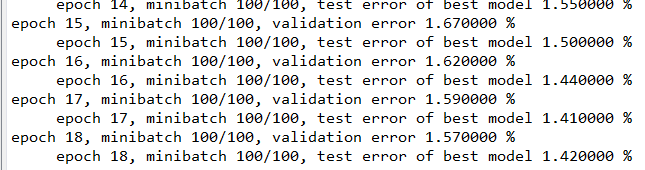1、http://blog.csdn.net/zouxy09/article/details/8775360/

2、http://www.deeplearning.net/tutorial/lenet.html#lenet

**********************作者：hjimce   时间：2015.8.6  联系QQ：1393852684   地址：http://blog.csdn.net/hjimce 转载请保留本行信息********************